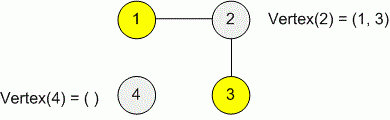Competitions

# Operations on graph

Create an undirected graph that supports the next operations:

1. AddEdge(u, v) - add to the graph an edge between the vertices (u, v);
2. Vertex(u) - print the list of vertices, adjacent with the vertex u.

There is no loops and multiple edges in the graph.

#### Input

The first line contains the number of vertices in a graph n (1n105). The next line contains the number of operations k (0k106). Each next line describes the operation in format: "1 <number> <number>" or "2 <number>", representing respectively the operation AddEdge(u, v) and Vertex(u).

It is guaranteed that the total amount of numbers to be printed during all operations Vertex, does not exceed 2 * 105.

#### Output

For each operation Vertex print in a separate line the list of adjacent vertices for a given vertex. The vertices in a list can be printed in any order. If some vertex hasn't adjacent vertices, print the empty line.Time limit 3 seconds
Memory limit 128 MiB
Input example #1
4
4
1 1 2
1 2 3
2 4
2 2

Output example #1
1 3